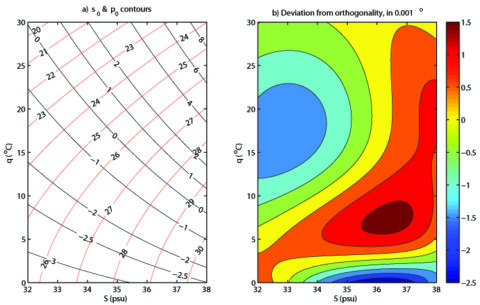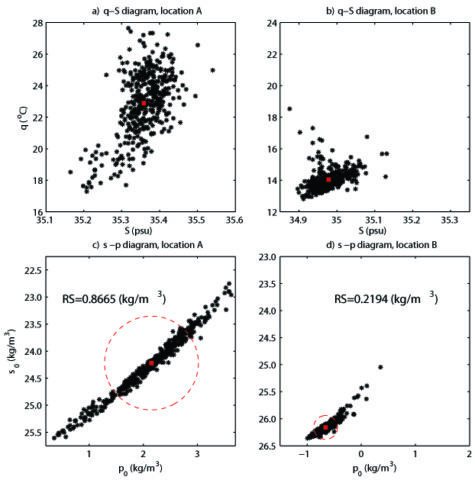# Potential Spicity: From Abstract Theory to Practical Application

A method for estimating potential spicity, a thermodynamic variable in oceanography, provides a new way to describe contrasts in watermass properties.Ocean water, seen from below the surface, with ripples, air bubbles, and sunlight visible.

By

The characteristics of seawater can be measured by different properties such as temperature, salinity, pressure and density. All are important variables for understanding the dynamics of ocean circulation. Another thermodynamic variable is “spiciness.” To date, spiciness has only been used as an abstract theoretical concept, but new developments have the potential for practical application in physical oceanography.

Potential density (σ) is a widely used concept in oceanography, since large-scale water movements largely follow surfaces of constant potential density, known as “isopycnal surfaces.” However, what happens perpendicular to the isopycnal surface is also of great interest and scientific importance.

It has long been a goal of oceanographers to develop a mathematical function to calculate an orthogonal coordinate between the isopycnal surface and the perpendicular property, which is a theoretical concept known as spiciness. A major challenge in this mathematical task is that a perfect spiciness variable does not exist due to the nonlinear nature of the equation of the state of seawater. Despite several attempts in previous works, no spiciness variable satisfying the orthogonality constraint against potential density has been found.

In a paper recently published in Journal of Geophysical Research: Oceans, Huang et al.  attempt to make progress with this challenge. They designed a two-step linearized algorithm to estimate the potential spicity (π), which minimizes the root mean square of angle deviation from the gradient of potential density (σ), using the method of least squares.

They evaluate potential spicity in a potential temperature (θ)–salinity (S) domain, conditions that cover most seawater in the global open ocean. The deviation of the angle between potential spicity and potential density from orthogonality is on the order of 10-4 degree, which is much smaller than the errors in contemporary in-situ temperature and salinity measurements. Given the lack of a perfect spiciness variable, it is impressive and surprising how the potential spicity accurately captures the desired orthogonality.The left panel shows contours of potential spicity (π) and potential density (σ) in the potential temperature (θ)–salinity (S) diagram. The right panel shows deviation of the angle between π and σ from orthogonality. Credit: Huang et al. , Figure 5Data for two locations both at 0.5˚S and a depth of 145 meters, with A (left panels) being at 179.5˚W in the western Pacific and above the main thermocline, and B (right panels) being at 100.5˚W in the far eastern Pacific and below the thermocline. The black dots show properties measured from 1980 to 2015 with the data presented both in the θ-S diagram (top panels) and the σ-π diagram (bottom panels). The red dots represent the mean values and the red circles mark the radius of the signals in both locations. Credit: Huang et al. , Figure 9Since potential spicity and potential density form a new (almost) orthogonal curvilinear coordinate, this σ-π diagram could become an alternative tool for describing the contrasts in watermass properties alongside the classical θ-S diagram.

Particularly, since the two axes of the σ-π diagram share the same unit and the coordinate is orthogonal, the distance between two water parcels can be defined.

Furthermore, the radius of a signal carried by a watermass can be defined as the root mean square of the watermass properties in the σ-π diagram, which is a potentially useful indicator to the sensitivity of a watermass to an external forcing, such as the El Niño Southern Oscillation (ENSO).

In sum, thanks to the work of Huang et al. , we now have two coordinate systems, θ-S and σ-π, available for watermass analysis. The introduction of potential spicity, along with an algorithm with high accuracy, brings the abstract theoretical concept of spiciness to a possible practical application.

So far, the use and significance of the potential spicity has not been fully explored. There are also concerns about the nature of the orthogonality condition and the inherent non-passivity of various measures of spiciness. Nevertheless, potential spicity is expected to be a useful tool for research in oceanography and we will hopefully see more studies on this topic in the future.

—Lei Zhou (email: [email protected]), Editor, JGR: Oceans, and Institute of Oceanography, Shanghai Jiao Tong University, China

Citation: Zhou, L. (2018), Potential spicity: From abstract theory to practical application, Eos, 99, https://doi.org/10.1029/2018EO108945. Published on 16 November 2018.
Text © 2018. The authors. CC BY-NC-ND 3.0
Except where otherwise noted, images are subject to copyright. Any reuse without express permission from the copyright owner is prohibited.